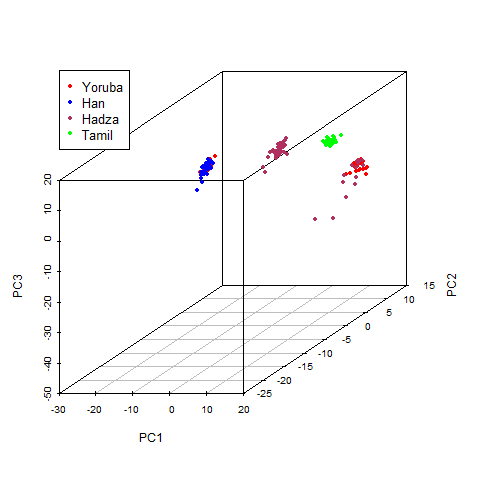# PCA of multilocus genotypes in R

An earlier post from Mark Christie showed up on my feed on calculating allele frequencies from genotypic data in R, and I wanted to put together a quick tutorial on making PCA (Principal Components Analysis) plots using genotypes. I used the genotype data published by Tishkoff et al. (2009) for this example, but it should work for any generic genotype format, as long as it’s stored as a table/matrix. As an example, let’s try plotting the first three principal components for the Yoruba, Hadza, Han, and Tamil populations.PCA (first 3 PC’s shown) of genotypes from 4 populations (Hadza, Yoruba, Han, and Tamil) using genotypic data from Tishkoff et al. (2009)

```install.packages(“scatterplot3d”)
install.packages(“rgl”)
library(scatterplot3d)
library(rgl)
tishkoff.pca<-prcomp(tishkoff[,4:1000],
center=TRUE,scale.=TRUE)
pcs123<-tishkoff.pca\$x[,1:3]
yoruba<-grep(“Yoruba”,tishkoff\$population.name)
han<-grep(“Han”,tishkoff\$population.name)
tamil<-grep(“Tamil”,tishkoff\$population.name)
p<-scatterplot3d(pcs123[yoruba,1],pcs123[yoruba,2]
,pcs123[yoruba,3],color=c(“red”),
pch=20,xlab=“PC1”,ylab=“PC2”,zlab="PC3",
xlim=c(min(pcs123[,1]),max(pcs123[,1])),
ylim=c(min(pcs123[,2]),max(pcs123[,2])),
zlim=c(min(pcs123[,3]),max(pcs123[,3])))
p\$points3d(pcs123[han,1],pcs123[han,2],
pcs123[han,3],col=c(“blue”),pch=20)
p\$points3d(pcs123[tamil,1],pcs123[tamil,2],
pcs123[tamil,3],col=c(“green”),pch=20)
legend(p\$xyz.convert(-80, 20, -10),
col= c(“red”,“blue”, “maroon”, “green”),
bg=“white”, pch=c(20,20,20,20), yjust=0,
legend = c(“Yoruba”, “Han”, “Hadza”, “Tamil”),
cex = 1.1)
```

And voila! I bet there are plenty of more complex packages (in R and other tools) that would help make similar plots – for instance, see adegenet, and SNPRelate, but do try my script out and leave your suggestions/comments below!